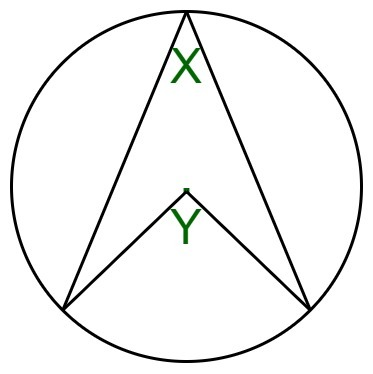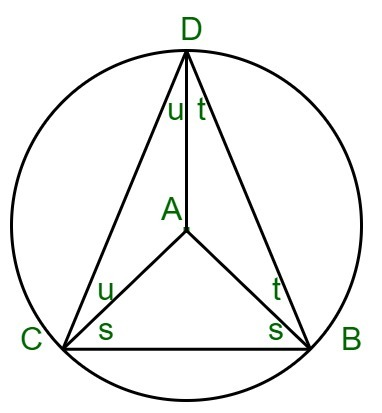# Angle subtended by an arc at the centre of a circle

Given the angle subtended by an arc at the circle circumference X, the task is to find the angle subtended by an arc at the centre of a circle.

For eg in the below given image, you are given angle X and you have to find angle Y.Examples:

Input: X = 30
Output: 60

Input: X = 90
Output: 180

## Recommended: Please try your approach on {IDE} first, before moving on to the solution.

Approach:• When we draw the radius AD and the chord CB, we get three small triangles.
• The three triangles ABC, ADB and ACD are isosceles as AB, AC and AD are radiuses of the circle.
• So in each of these triangles, the two acute angles (s, t and u) in each are equal.
• From the diagram, we can see
`D = t + u (i)`
• In triangle ABC,
```s + s + A = 180 (angles in triangle)
ie, A = 180 - 2s  (ii)
```
• In triangle BCD,
```(t + s) + (s + u) + (u + t) = 180 (angles in triangle again)
so 2s + 2t + 2u = 180
ie 2t + 2u = 180 - 2s (iii)```
• So
`A = 2t + 2u = 2D from (i), (ii)  and (iii)`
• Hence Proved that ‘the angle at the centre is twice the angle at the circumference‘.

Below is the implementation of the above approach:

## C++

 `// C++ implementation of the approach ` ` `  `#include ` `using` `namespace` `std; ` ` `  `// Function to find Angle ` `// subtended by an arc ` `// at the centre of a circle ` `int` `angle(``int` `n) ` `{ ` `    ``return` `2 * n; ` `} ` ` `  `// Driver code ` `int` `main() ` `{ ` `    ``int` `n = 30; ` `    ``cout << angle(n); ` ` `  `    ``return` `0; ` `} `

## Java

 `// Java implementation of the approach ` `import` `java.io.*; ` ` `  `class` `GFG ` `{ ` `     `  `// Function to find Angle subtended  ` `// by an arc at the centre of a circle ` `static` `int` `angle(``int` `n) ` `{ ` `    ``return` `2` `* n; ` `} ` ` `  `// Driver code ` `public` `static` `void` `main (String[] args) ` `{ ` `    ``int` `n = ``30``; ` `    ``System.out.println(angle(n)); ` `} ` `} ` ` `  `// This code is contributed by ajit. `

## Python3

 `# Python3 implementation of the approach ` ` `  `# Function to find Angle ` `# subtended by an arc ` `# at the centre of a circle ` `def` `angle(n): ` `    ``return` `2` `*` `n ` ` `  `# Driver code ` `n ``=` `30` `print``(angle(n)) ` ` `  `# This code is contributed by Mohit Kumar `

## C#

 `// C# implementation of the approach ` `using` `System; ` ` `  `class` `GFG ` `{ ` `     `  `// Function to find Angle subtended  ` `// by an arc at the centre of a circle ` `static` `int` `angle(``int` `n) ` `{ ` `    ``return` `2 * n; ` `} ` ` `  `// Driver code ` `public` `static` `void` `Main() ` `{ ` `    ``int` `n = 30; ` `    ``Console.Write(angle(n)); ` `} ` `} ` ` `  `// This code is contributed by Akanksha_Rai  `

Output:

```60
```

Time Complexity: O(1)

Attention reader! Don’t stop learning now. Get hold of all the important DSA concepts with the DSA Self Paced Course at a student-friendly price and become industry ready.

My Personal Notes arrow_drop_upCheck out this Author's contributed articles.

If you like GeeksforGeeks and would like to contribute, you can also write an article using contribute.geeksforgeeks.org or mail your article to contribute@geeksforgeeks.org. See your article appearing on the GeeksforGeeks main page and help other Geeks.

Please Improve this article if you find anything incorrect by clicking on the "Improve Article" button below.